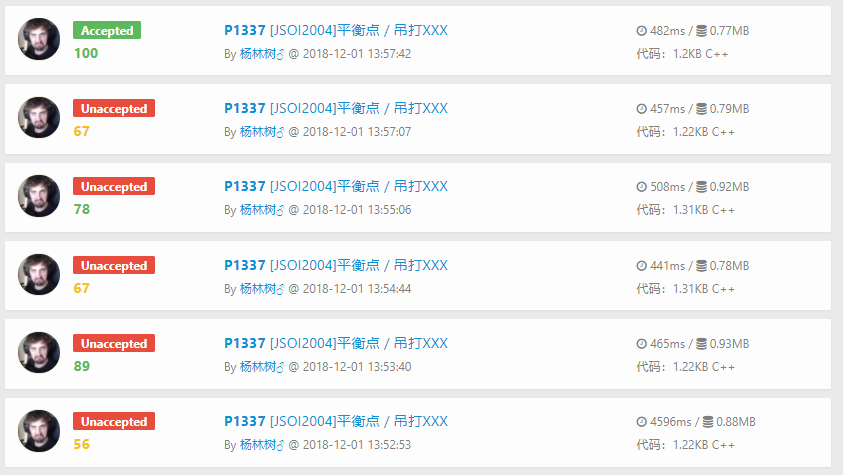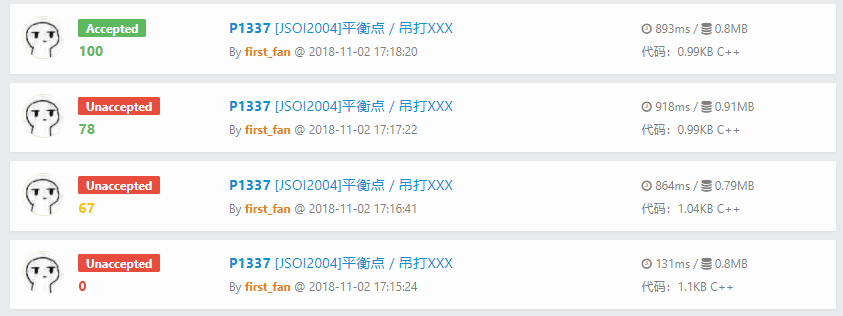·算法过程

首先就是模拟退火的随机设置自然就是把随机数种子设置好，别忘了先洗脸，然后通过模拟退火原理设置好转移概率表达式:

bool accept(int del)
{
return ((del>0)||exp(-del/T) > (double)rand()/RAND_MAX);
} //转移概率表达式

·偏移过程

这就是上述问题的用武之地了: 以一定的概率不选当前最优解中的那个物品，从而衍生出另一种方案，如果比刚刚的更好，那么说明运气很好，跳到了一个更优解范围附近

if(accept(dE))
{//以上述概率发生转移
if(vis[a])
{
vis[a] = false;
tot -= v[a];
}//在当前物品已选的情况下放弃选用当前物品
else
{
if(tot + v[a] > V)
{
continue;
}
vis[a] = true;
tot += v[a];
}//在当前物品未选的情况下尝试选用当前物品
}

·退火步骤

while(T > 1e-14) {
ans=max(ans,tot); //维护最优答案，以防非酋情况发生
a = rd; //进行随机
int dE = v[a];
if(vis[a])
{
dE *= -1; //产生能量差
}
if(accept(dE))
{//发生转移
if(vis[a])
{
vis[a] = false;
tot -= v[a];
}
else
{
if(tot + v[a] > V)
{
continue;
}
vis[a] = true;
tot += v[a];
}
}
T *= delta; //降温
}

番外篇: 论欧皇的养成

iewqufhiwefdiweduiqwediuqedwedjewui

(被某个非酋网友摁在键盘上打/笑哭)这是我的↓first_fan

Just Do It,But Not Just Do It.